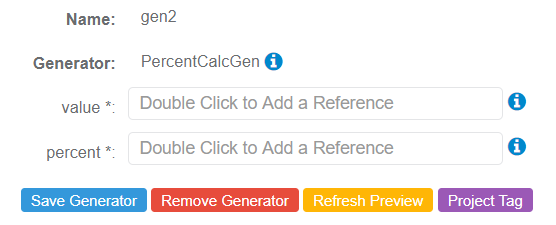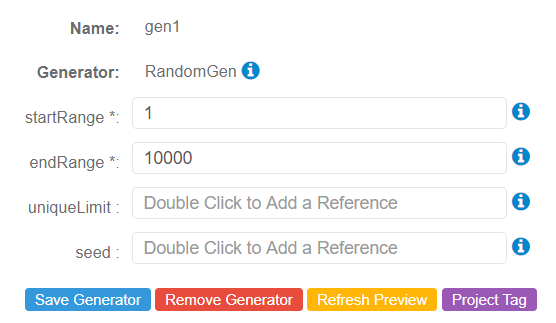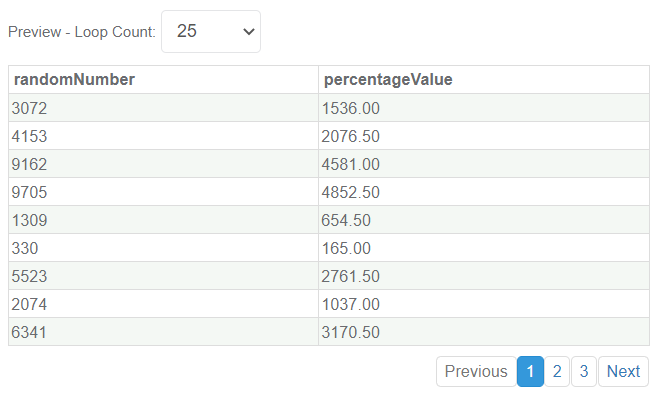# Description

The PercentCalcGen Generator calculates the percentage of the specified amount.

## Parameters

The following parameters may be configured for the PercentCalcGen Generator. Items with an asterisk* are required.

• value* - The initial value used to calculate the percentage.

• percent* - The percentage the specified amount will be multiplied by to calculate the new value.## Example 1: Generate Random Number from 1 to 1000 and Calculate 50% of the Value

The RandomGen Generator has been linked to generate a random number between 1 and 1000.

The PercentCalcGen Generator will then calculate 50 percent or half of that value.RandomGen Generator Configuration:## Sample Output